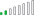cancel
Showing results for
Did you mean:Frequent Visitor

## Filter duplicate values with more arguments

Hi,

I need to add another filter argument in my dax formular.

Right now, I have the following:

Duplicate Cust. Ref = CALCULATE(

COUNT(

'ABC'[DEF]

),

Filter(

'ABC',

'ABC'[DEF]=EARLIER('ABC'[DEF)

)

)

Now I need to include also the column "ABC[XYZ]" as a second argument to filter. So I only need the count when both arguments are true.

Any help how I can achieve this?

Thanks

Alexander

1 ACCEPTED SOLUTIONCommunity Support

Hi @oldrunner ,

The EARLIER function is usually used in calculated columns, please use the MAX or SELECTEDVALUE function in the measure, like this:

``````Duplicate Cust. Ref =
CALCULATE (
COUNT ( 'ABC'[DEF] ),
Filter ( 'ABC', 'ABC'[DEF] = MAX ( 'ABC'[DEF] ) )
)``````If the problem is still not resolved, please provide detailed error information or the expected result you expect. Let me know immediately, looking forward to your reply.
Best Regards,
Winniz
If this post helps, then please consider Accept it as the solution to help the other members find it more quickly.

5 REPLIES 5Community Support

Hi @oldrunner ,

The EARLIER function is usually used in calculated columns, please use the MAX or SELECTEDVALUE function in the measure, like this:

``````Duplicate Cust. Ref =
CALCULATE (
COUNT ( 'ABC'[DEF] ),
Filter ( 'ABC', 'ABC'[DEF] = MAX ( 'ABC'[DEF] ) )
)``````If the problem is still not resolved, please provide detailed error information or the expected result you expect. Let me know immediately, looking forward to your reply.
Best Regards,
Winniz
If this post helps, then please consider Accept it as the solution to help the other members find it more quickly.Frequent Visitor

I get the message that "earlier" functions cannot be nested. So, unfortunately, this did not work.
Maybe my question was not quite correct. I need to filter on two different columns and it should only count when both (or more) arguments are true. So for example the customer name and the purchase order number (as different customers might have the same purchase order number).
Thanks

AlexFrequent Visitor

Thanks.
I get the message that earlier functions cannot be nested. Maybe my question was not quite correct. I need to filter on two different columns and it should only count when both (or more) arguments are true. So for example the customer name and the purchase order number (as different customers might have the same purchase order number).
Thanks

AlexSuper User

@oldrunner , Check the solution by @darshaningale . If that do not work try

Duplicate Cust. Ref = CALCULATE(
COUNT(
'ABC'[DEF]
),
Filter(
'ABC',
'ABC'[DEF]=EARLIER('ABC'[DEF)
&& ABC[XYZ] = earlier(ABC[XYZ])
)

)Resolver II

Duplicate Cust. Ref = CALCULATE(

COUNT(

'ABC'[DEF]

),

Filter(

'ABC',

'ABC'[DEF]=EARLIER('ABC'[DEF) && ABC[XYZ] ='something'

)

)

Regards
DI

Did I answer your question? Mark my post as a solution, this will help others!
Kudos are also welcome.